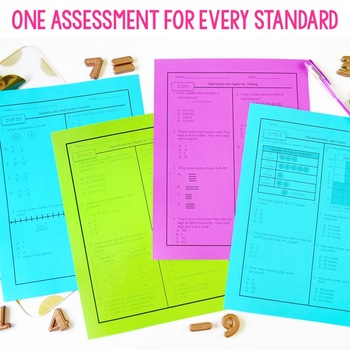# 3rd Grade Math Assessments Test Prep | Editable & Digital3rd, Homeschool
Subjects
Standards
Resource Type
Formats Included
• Zip
•Google Apps™
Pages
31 pagesThe Teacher-Author indicated this resource includes assets from Google Workspace (e.g. docs, slides, etc.).

### Description

Get a multiple choice assessment for every Common Core Math Standard! Check out the preview for two FREE pages!

You can prepare your students for their upcoming standardized tests with these multiple choice Common Core math assessments for 3rd grade. In this file, there is a one page assessment for each of the Common Core math standards. The answer choices were selected intentionally for common errors students make in their computation and math reasoning.

More Test Prep Products

Super Testers-Weekly Math Practice

Math Constructed Response

Spring Centers for Test Prep

Total Pages
31 pages
Included
Teaching Duration
N/A
Report this Resource to TpT
Reported resources will be reviewed by our team. Report this resource to let us know if this resource violates TpT’s content guidelines.

### Standards

to see state-specific standards (only available in the US).
Tell and write time to the nearest minute and measure time intervals in minutes. Solve word problems involving addition and subtraction of time intervals in minutes, e.g., by representing the problem on a number line diagram.
Measure and estimate liquid volumes and masses of objects using standard units of grams (g), kilograms (kg), and liters (l). Add, subtract, multiply, or divide to solve one-step word problems involving masses or volumes that are given in the same units, e.g., by using drawings (such as a beaker with a measurement scale) to represent the problem.
Draw a scaled picture graph and a scaled bar graph to represent a data set with several categories. Solve one- and two-step “how many more” and “how many less” problems using information presented in scaled bar graphs. For example, draw a bar graph in which each square in the bar graph might represent 5 pets.
Generate measurement data by measuring lengths using rulers marked with halves and fourths of an inch. Show the data by making a line plot, where the horizontal scale is marked off in appropriate units-whole numbers, halves, or quarters.
Recognize area as an attribute of plane figures and understand concepts of area measurement.